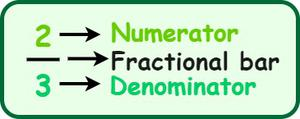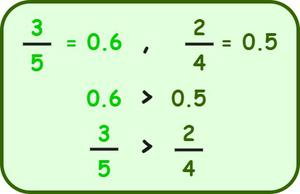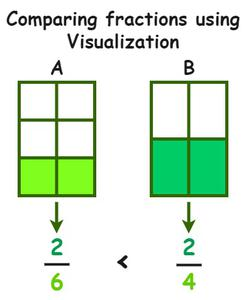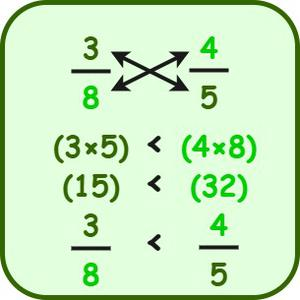Open in App
Not now

# Comparing Fractions – Definition, Methods, Examples, FAQs

• Last Updated : 22 Aug, 2022

Comparison of fractions” refers to the determination of the larger and the smaller fraction within a given set of fractions. While comparing fractions, a set of rules is followed to compare the numerator and denominator of a fraction, where the numerator is the number above the fractional bar and the denominator is the number below the fractional bar. We can determine the greater and smaller fractions by comparing any two fractions. Fractions can be compared even if they have different numerators and denominators. To understand the concept better, let’s go over the various ways to compare fractions.

## Fraction

Before going through the concept of comparing two fractions, let us recall what a fraction is. A fraction is defined as a part of a whole that consists of two parts: the numerator and the denominator, where the numerator is the number above the fractional bar and the denominator is the number below the fractional bar.## How to compare two fractions in numbers?

In order to determine which of the two fractions is larger or smaller, one has to compare them. Based on the numerator and the denominator and the kind of fractions given there are different methods and rules to compare fractions. They are:

1. Comparing Fractions with Same Denominators
2. Comparing Fractions With Unlike Denominators
3. Comparing Fractions using the Decimal Method
4. Comparing Fractions using Visualization
5. Comparing Fractions using the Cross Multiplication method

### Comparing Fractions with Same Denominators

It is easy to find the greater or smaller fraction when the fractions have the same denominators. When comparing fractions, check whether the denominators are the same or not. If the denominators are equal, then the fraction with the bigger numerators is the bigger fraction. The fractions are equal if the numerators and denominators of both fractions are equal.

Example: Compare: 5/12 and 17/12.

Solution:

Step 1: First, observe the denominators of the given fractions, i.e., 5/12 and 17/12. Here, the denominators are the same for both fractions.

Step 2: Now, compare the numerators of the given fractions. We can observe that 17 > 5.

Step 3: We know that the fraction with the larger numerator is larger. Hence, 5/12 < 17/12.

### Comparing Fractions With Unlike Denominators

To compare fractions with unlike denominators, we have to convert them to like denominators for which we have to find the Least Common Multiple (LCM) of the denominators. As the denominators are made equal, we can compare the fractions with ease.

Example: Compare: 1/4 and 2/3.

Solution:

Step 1: First, observe the denominators of the given fractions, i.e., 1/4 and 2/3. Since the denominators are different make them equal by finding the LCM of 4 and 3. LCM(4,3) = 12.

Step 2: Now, let us convert the given fraction in such a way that they have the same denominators. So, multiply the first fraction with 3/3, i.e., 1/4 × 3/3 = 4/12.

Step 3: Similarly, multiply the second fraction with 4/4, i.e., 2/3 × 4/4 = 8/12. Thus, the first fraction becomes 4/12 and the other becomes 8/12.

Step 4: Compare the obtained new fractions, i.e., 4/12 and 8/12. As the denominators are the same, we will compare the numerators. We can observe that 4 < 8.

Step 5: The fraction that has a large numerator is the larger fraction. So, 8/12 > 4/12. So, 1/4 > 2/3.

Note: Make a note that if the given fractions have the same numerators and different denominators, then we can compare them easily by looking at their denominators. The fraction that has a smaller denominator has a greater value, while the fraction that has a larger denominator has a smaller value. For example 6/2 > 6/5.

### Comparing Fractions using the Decimal Method

In this method, one can compare fractions by finding the decimal values of the fractions and comparing them. For this, divide the numerator by the denominator, and thus the fraction is converted into a decimal. Finally, compare their decimal values. Let us understand this by going through an example.

Example: Compare 3/5 and 2/4.

Solution:Step 1: To write 3/5 and 2/4 in decimals, divide the numerator by the denominator. Divide 3 by 5, and 2 by 4.

Step 2: The obtained decimal values are 0.6 and 0.5.

Step 3: Finally, compare the decimal values. 0.6 > 0.5. The fraction that has a larger decimal value would be larger. Hence, 3/5 > 2/4.

### Comparing Fractions using Visualization

Compared to any other method, comparing fractions using visualization is easier. Make two boxes such that the length and width of both are the same. The figure is given below shows models A and B, which represent two fractions. Then divide each model into equal parts equivalent to their respective denominators. Now, we can easily find that 2/6 < 2/4, as the 2/4 covers a larger shaded area compared to the 2/6. The smaller fraction occupies a lesser area of the same whole, while the larger fraction occupies a larger area of the same whole.### Comparing Fractions using the Cross Multiplication method

To compare fractions using cross multiplication, we have to multiply the numerator of one fraction with the other fraction’s denominator. Let us understand this by going through an example.

Example: Compare 3/8 and 4/5.

Observe the figure given below which explains the concept of cross multiplication better.Step 1: Make a note that when we are performing cross multiplication to compare two fractions, we have to multiply the numerator of the first fraction with the second fraction’s denominator. We have to write the product on the side of the selected numerator. Here, the product is 3 × 5 = 15, which we write near the first fraction.

Step 2: Similarly, when multiplying the second fraction’s numerator with the first fraction’s denominator, we have to write the product next to the second fraction. Here, the product is 4 × 8 = 32, which we will write near the second fraction.

Step 3: Now, compare both products, i.e., 15 and 32. Since 15 < 32, the respective fractions can be easily compared, i.e., 3/8 < 4/5. Hence, 3/8 < 4/5.

## Solved Examples on Comparison of Fractions

Example 1: Which of the following fractions is larger: 6/11 or 8/15?

Solution:

Given fractions: 6/11 and 8/15

The denominators of the given fractions are different. So, find out the LCM of the denominators, i.e., LCM(11, 15) = 165.

Now, multiply 6/11 with 15/15 and 8/15 with 11.

6/11 × 15/15 = 90/165

8/15 × 11/11 = 88/165

Compare the numerators now, as the denominators are the same.

So, 90 > 88, i.e., 90/165 > 88/165.

Hence, 6/11 > 8/15, i.e., 6/11 is the larger fraction.

Example 2: Which of the given fractions is smaller: 13/85 or 21/85?

Solution:

Given fractions: 13/85 and 21/85.

The denominators of the given fractions are the same. So, compare the numerators of the given fractions.

13 < 21.

So, 13/85 < 21/85.

Hence, 13/85 is the smaller fraction.

Example 3: Compare the fractions 4/25 and 33/100.

Solution:

To compare the given fractions, find their decimal values. So, divide 4 by 25 and 33 by 100.

4/25 = 0.16

33/100 = 0.33

From the decimal values, we can conclude that 0.33 > 0.16. So, 33/100 is greater than 4/25.

Therefore, 33/100 is greater than 4/25.

Example 4: Mrunal was asked to prove that the given fractions are equal: 30/90 and 25/75. Can you prove the given statement using the LCM method?

Solution:

Given fractions: 30/90 and 25/75.

The denominators of the given fractions are different. So, find out the LCM of the denominators, i.e., LCM(90, 75) = 450.

Now, multiply 30/90 with 5/5 and 25/75 with 6/6.

30/90 × 5/5 = 150/450

25/75 × 6/6 = 150/450

Compare the numerators now, as the denominators are the same.

So, 150 = 150, i.e., 150/450 = 150/450.

Thus, 30/90 = 25/75, i.e., both the given fractions are equal.

Hence, proved.

Example 5: Which of the following fractions is larger: 27/41 or 27/67?

Solution:

Given fractions: 27/41 and 27/67.

Here, the numerators of both fractions are the same but the denominators are different.

We know that the fraction that has a smaller denominator has a greater value, while the fraction that has a larger denominator has a smaller value.

Here, 41 < 67.

So, 27/41 > 27/67

Therefore, 27/41 is the larger fraction.

## FAQs on Comparing Fractions

Question 1: What is meant by comparing fractions?

“Comparison of fractions” refers to the determination of the larger and the smaller fraction within a given set of fractions. We can determine the greater, smaller, or equivalent fractions by comparing any two fractions. Based on the numerator and the denominator and the kind of fractions given there are different methods and rules to compare fractions.

Question 2: What is the rule for comparing fractions that have the same denominator?

If the given set of fractions has the same denominator, then the fraction that has a smaller numerator is the smaller fraction, and the fraction that has a larger numerator is the larger fraction. If the numerators are equal, then the fractions are considered to be equivalent fractions. For example, if we have to compare 2/3 and 5/3, we just need to check their numerators and compare them. Since 2 < 5, so 2/3 < 5/3.

Question 3: What is the Rule when Comparing Fractions with the Same Numerator?

If the given set of fractions has the same numerator, then the fraction that has a smaller denominator is the larger fraction, and the fraction that has a larger denominator is the smaller fraction. For example, if we have to compare 4/3 and 4/9, we just need to check their denominators and compare them. Now, out of 4/3 and 4/9, the fraction with the smaller denominator is 4/3. Hence, 4/3 is the larger fraction of the given fractions.

Question 4: What are Equivalent Fractions?

Equivalent fractions are those that have distinct numerators and denominators but have the same values when simplified. For example, 3/12 and 5/20 are equivalent fractions as both of them are equal to 1/4 when simplified.

Question 5: How to Compare Fractions with Different Denominators?

To compare fractions with unlike denominators, we have to convert them to like denominators for which we have to find the Least Common Multiple (LCM) of the denominators. As the denominators are made equal, we can compare the fractions with ease. For example, let us compare 1/4 and 2/3.

Step 1: First, observe the denominators of the given fractions, i.e., 1/4 and 2/3. Since the denominators are different make them equal by finding the LCM of 4 and 3. LCM(4,3) = 12.

Step 2: Now, let us convert the given fraction in such a way that they have the same denominators. So, multiply the first fraction with 3/3, i.e., 1/4 × 3/3 = 4/12.

Step 3: Similarly, multiply the second fraction with 4/4, i.e., 2/3 × 4/4 = 8/12. Thus, the first fraction becomes 4/12 and the other becomes 8/12.

Step 4: Compare the obtained new fractions, i.e., 4/12 and 8/12. As the denominators are the same, we will compare the numerators. We can observe that 4 < 8.

Step 5: The fraction that has a large numerator is the larger fraction. So, 8/12 > 4/12. So, 1/4 > 2/3.

My Personal Notes arrow_drop_up
Related Articles